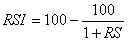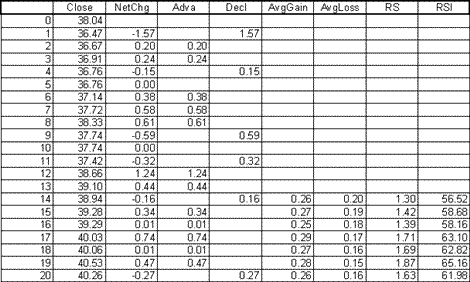Chart Pro online
User name (email):

 Recently used

 Forex

 Indices

# Relative Strength Index (RSI)

Relative strength index (RSI) was introduced in 1978 by J. Welles Wilder in his book "New Concepts in Technical Trading Systems." It belongs to a group of leading indicators.

The formula for calculating relative strength index is:RS = average gain / average loss Average gain = [(previous average gain) (n-1) + current average gain] / n First average gain = total gain in the first n periods / n Average loss = [(previous average loss) (n-1) + current average loss] / n First average loss = total losses in the first n periods / n

If the current closing price is higher than the previous one, it is considered as gain. Average gain averages difference between closing prices. The average loss averages those differences where closing price is less than the previous one. But bear in mind that the average loss is a positive value.Relative Strength Index calculation

The most common value for n is 14. As n is lower oscillator has a higher amplitude.Relative strength index

Interpretation

As with any other oscilator, buying and selling signals are generated when value of relative strength index crosses defined boundaries. If RSI crosses above 70, value of security is overbought and selling signal is generated. If value crosses below 30, security is oversold and buying signal is generated.

Wilder has spotted a phenomenon on RSI chart which he named failure swing. Failure swing occurs when the value of RSI is greater than 70 and RSI fails to reach the previous top. If value of RSI drops below its previous bottom it is a signal that price reached its peak. For bottom failure swing opposite is true and it looks like mirrored top failure swing.

RSI can also be used for observation of divergence. So if price falls to new low, but relative strength index fails to reach new low, downward trend is losing its power and we could expect trend reversal in the near future. Similar goes for upward trend: if RSI fails to reach new high when price hits new high, upward trend is losing its power.

Some traders use line 50 to generate signals: if value of RSI crosses above 50 selling signal is generated and if it crosses below 50, buying signal is generated.

When the value of RSI is above level of 70 it is considered that a financial instrument is overbought, and when it is below 30, it is oversold. However, in strong trends often happens that this logic leads to generating of false signals. Thus, in strong upward trend when the RSI crosses above 70, it often happens that the price of financial instruments continues to grow for some time. That's way some traders generate selling signal when RSI crosses below 70, similar goes with buying signals and line of 30.

Example